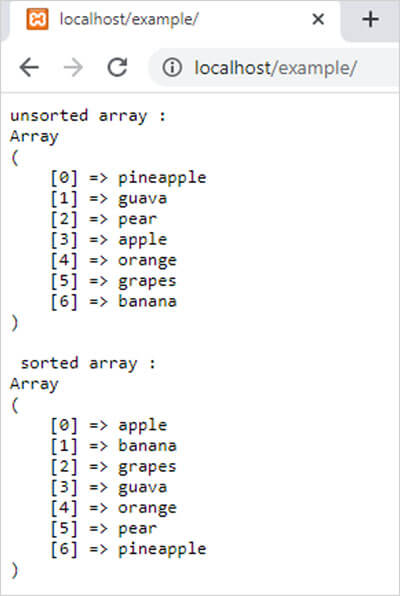PHP sort() function || PHP Sorting Arrays# PHP sort() function

1608

PHP sort() function is used to sort a PHP indexed array in ascending order. It is an inbuilt function of PHP.

NOTE: For descending order use rsort() PHP function.

Syntax:

sort(\$array, \$sorting_type)

here,

1. \$array is a PHP array. It is mandatory.

2. \$sorting_type is a numeric value that decides the type of comparing of array elements. It is optional. It has 6 possible values that specify 6 possible sorting orders.

• SORT_REGULAR: if a value is 0, then the function compares array elements normally.
• SORT_NUMERIC: if a value is 1, then the function compares array elements as a numeric value.
• SORT_STRING: if a value is 2, then the function compares array elements as a string.
• SORT_LOCALE_STRING: if a value is 3, then this function compares array elements as a string according to the current locale.
• SORT_NATURAL: if a value is 4, then the function compares array elements as a string with the use of natural ordering.
• SORT_FLAG_CASE: if a value is 5, then this function compares array elements according to value.

Example:

```<?php
\$arr1 = array("pineapple","guava","pear","apple","orange","grapes","banana");
echo "<pre>";
echo "unsorted array : <br>";
print_r(\$arr1);
sort(\$arr1);
echo "<br> sorted array : <br>";
print_r(\$arr1);
?>```

Output:Share:## Big Hosting Days

Upto 75@ OFF on Web Hosting

## Get a .COM for just \$6.98

Secure Domain for a Mini Price